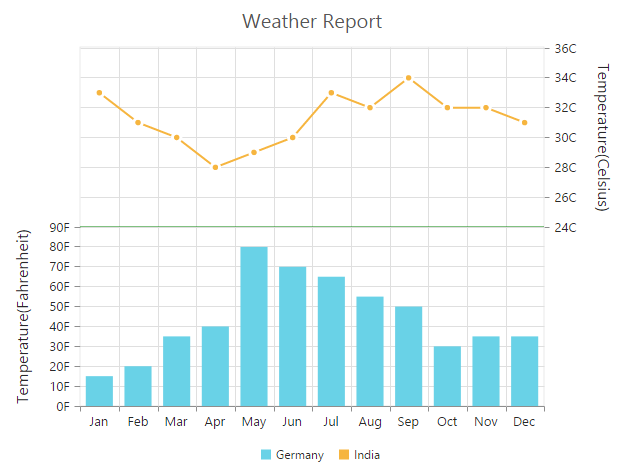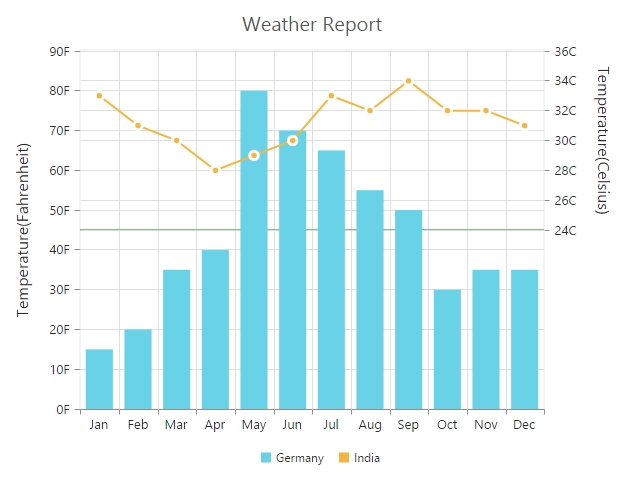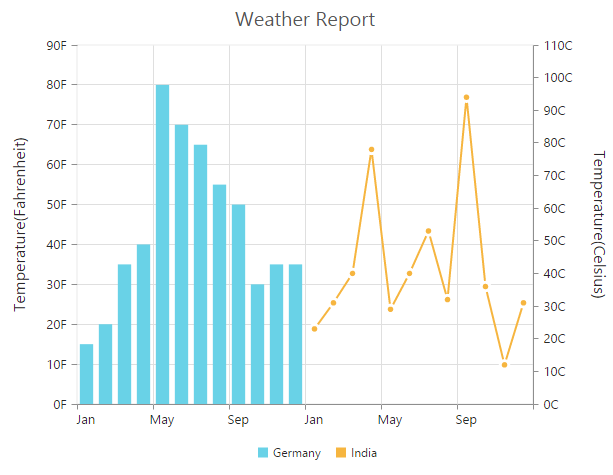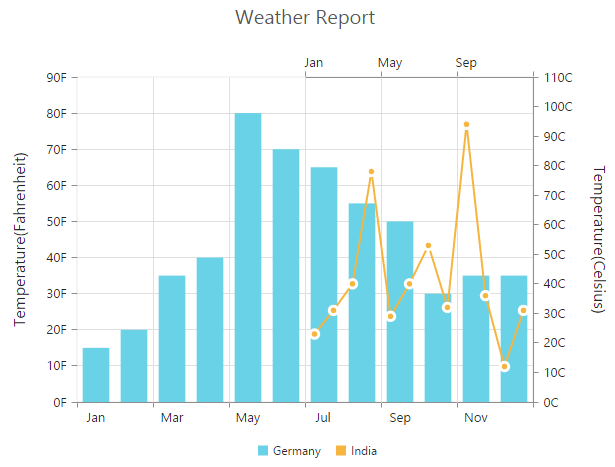# Multiple panes in EJ 1 Angular Chart

7 Jun 20174 minutes to read

Chart area can be divided into multiple panes using the `rowDefinitions` and `columnDefinitions` properties.

### Row Definitions

To split the chart area vertically into a number of rows, use `rowDefinitions` of the chart.

• You can allocate space for each row by using the `unit` option that determines whether the chart area should be split by percentage or pixels for the given `rowHeight` value of the rowDefinitions.

• To associate a vertical axis to a row, specify the rowDefinitions index value to the `rowIndex` property of the chart axis.

• To customize each row’s horizontal line, use `lineColor` and `lineWidth` property.

• TS
• ``````this.rowDefinitions = [{
//  Split first row of the chart area
unit: 'percentage',
lineColor: 'Gray',
rowHeight: 50,
linewidth: 0
}, {
//  Split second row of the chart area
unit: 'percentage',
lineColor: 'green',
rowHeight: 50,
linewidth: 0
}];
this.axes = [{
//Create secondary axis and bind it to second row of chart area
name: "yAxis1",
rowIndex: 1
}];``````
• HTML
• ``````<ej-chart id="chartcontainer" [rowDefinitions]="rowDefinitions" [axes]="axes">
<e-seriescollection>
<!--Binding vertical axis name-->
<e-series yAxisName="yAxis1">
</e-series>
</e-seriescollection>
</ej-chart>``````Row Span

For spanning the vertical axis along multiple panes vertically, you can use `rowSpan` property of axis.

• HTML
• ``````<ej-chart id="chartcontainer" [rowDefinitions]="rowDefinitions" [axes]="axes" [primaryYAxis.rows]=2>
<e-seriescollection>
<!--Binding vertical axis name-->
<e-series yAxisName="yAxis1">
</e-series>
</e-seriescollection>
</ej-chart>``````## Column Definitions

To split the chart area horizontally into a number of columns, use `columnDefinitions` of the chart.

• You can allocate space for each column by using the `unit` option that determines whether the chart area should be split by percentage or pixels for the given `columnWidth` value of the columnDefinitions.

• To associate a horizontal axis to a column, specify the columnDefinitions index value to the `columnIndex` property of the chart axis.

• TS
• ``````//  Splitting chart area into multiple columns
this.columnDefinitions = [{
//  Split first column of the chart area
unit: 'percentage',
columnWidth: 50,
}, {
//  Split second column of the chart area
unit: 'percentage',
columnWidth: 50,
}];

this.axes = [{
//Create secondary axis and bind it to second column of chart area
name: "xAxis1",
columnIndex: 1
}];``````
• HTML
• ``````<ej-chart id="chartcontainer" [columnDefinitions]="columnDefinitions" [axes]="axes">
<e-seriescollection>
<!--Binding horizontal axis name-->
<e-series xAxisName="xAxis1">
</e-series>
</e-seriescollection>
</ej-chart>``````Column Span

For spanning the horizontal axis along multiple panes horizontally, you can use `columnSpan` property of axis.

• HTML
• ``````<ej-chart id="chartcontainer" [columnDefinitions]="columnDefinitions" [axes]="axes" [primaryXAxis.columnSpan]=2>
<e-seriescollection>
<!--Binding horizontal axis name-->
<e-series xAxisName="xAxis1">
</e-series>
</e-seriescollection>
</ej-chart>``````